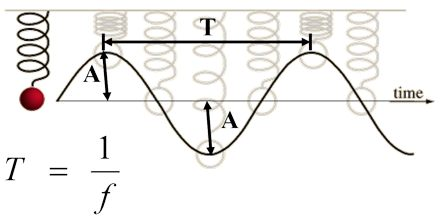Physics

# Graphical Analysis of Simple Harmonic MotionBasic objective of this lecture is to present on Graphical Analysis of Simple Harmonic Motion. Here briefly state the formula required for SHM and recognize equations and graphs which represent the variation of displacement, velocity and acceleration with time. Finally investigate simulations of mass-spring systems and the simple pendulum. Simple harmonic motion is typified by the motion of a mass on a spring when it is subject to the linear elastic restoring force given by Hooke’s Law. The motion is sinusoidal in time and demonstrates a single resonant frequency.